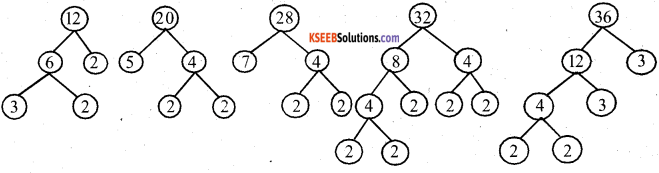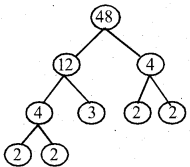# KSEEB Solutions for Class 5 Maths Chapter 4 Factors and Multiples

Students can Download Maths Chapter 4 Factors and Multiples Questions and Answers, Summary, Notes Pdf, KSEEB Solutions for Class 5 Maths helps you to revise the complete Karnataka State Board Syllabus and score more marks in your examinations.

## Karnataka State Syllabus Class 5 Maths Chapter 4 Factors and Multiples

### KSEEB Class 5 Maths Factors and Multiples Ex 4.1

What is the factor of 50? The factors of 50 are 1, 2, 5,10, 25, …

Question 1.
Circle the multiples of 4 with blue colour, cross the multiples of 6 with red colour and underline the multiples of 9 with a pencil.4, 8, 12, 16, 20, 24, 28, 32, 36, 40, 44, 48, 52, 56, 60, 64, 68, 72, 76, 80, 84, 88, 92, 96 & 100 → Multiples of 4
6, 12, 18, 24, 30, 36, 42, 48, 54, 60, 66, 72, 78, 84, 90 & 96 → Multiples of 6
9, 18, 27, 36, 45, 54, 63, 72, 81, 90 & 99 → Multiples of 9Question 2.
Circle the multiples of 7 in the following numbers.
7, 13, 14, 21, 22, 35, 36, 42 and 45
7, 14, 21, 35, 42

Question 3.
Circle the multiples of 12 in the following numbers.
6,12,18, 24, 30, 36, 42, 48, 54, 60, 66, 72
12, 24, 36, 48, 60, 72Question 4.
Write the multiples of 2 between the numbers 50 and 60.
52, 54, 56, 58
G : 52/2 = 26

Question 5.
Write the multiples of 15 between the numbers 50 and 100.
60, 75, 90
G : 15 × 4 = 60

Question 6.
Write five multiples of the following numbers.
5 multiples of 15 are 15, 30, 45, 60 & 75.
5 multiples of 17 are 17, 34, 51, 68 & 85.
5 multiples of 19 are 19, 38, 57, 76 & 95
5 multiples of 23 are 23, 46, 69, 92 & 115.The factors of 24 are 1, 2, 3, 4, 6, 8, 12 and 24! 24 has 8 factors in total.

Question 7.
Find which of the following numbers are factors of 24?
1, 2, 3, 4, 5, 6, 7, 8, 9, 10, 12, 14, 16, 18, 20, 22 ಮತ್ತು 24
24 – 1 × 24
24 – 4 × 6
24 – 2 × 12
24 – 3 × 8
1, 2, 3, 4, 6, 8, 12 & 24

Question 8.
Write any two factors of the following numbers.
6, 18, 28, 36, 42, 48
Any two factors of 6 are 2 & 3
Any two factors of 28 are 2 & 14
Any two factors of 42 are 2 & 21
Any two factors of 18 are 2 & 9
Any two factors of 36 are 3 & 12
Any two factors of 48 are 2 & 24Factoring Calculator will help you find the factors 18 i.e. 1, 2, 3, 6, 9, 18 the numbers that when divided results in a whole number and a zero remainder.

Question 9.
Write all the factors of the following numbers.
9, 13, 20, 26, 40
All the factors of 9 are 1,3 & 9 All the factors of 13 are 1 & 13 All the factors of 20 are 1, 2, 4, 5, 10 & 20
20 = 1 × 20
2 × 10 = 20
4 × 5 = 20
5 × 4 = 20
10 × 2 = 20
20 × 1 = 20
All the factors of 26 are 1 ,2, 13 & 26
All the factors of 40 are 1, 2, 4, 5, 8, 10, 20 & 40Question 10.
Write the factor tree for the following numbers:Prime factors of 84 are 2×2, 3, 7.

Question 11.
Complete the following factor tree by writing missing numbers.(i)(ii)The prime factors of 38 are 2 and 19.error: Content is protected !!